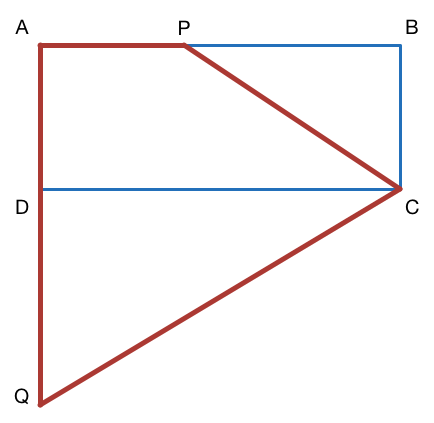Consider a rectangle which has a diagonal of length 6. If $P$ is a point on side $AB$ such that $\lvert\overline{AP}\rvert=\lvert\overline{AD}\rvert,$ and $Q$ is a point on the extension of side $AD$ such that $\lvert\overline{AQ}\rvert=\lvert\overline{AB}\rvert,$ what is the area of the quadrilateral $APCQ?$